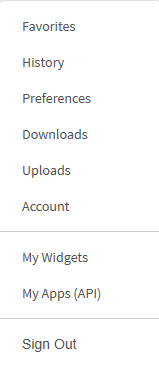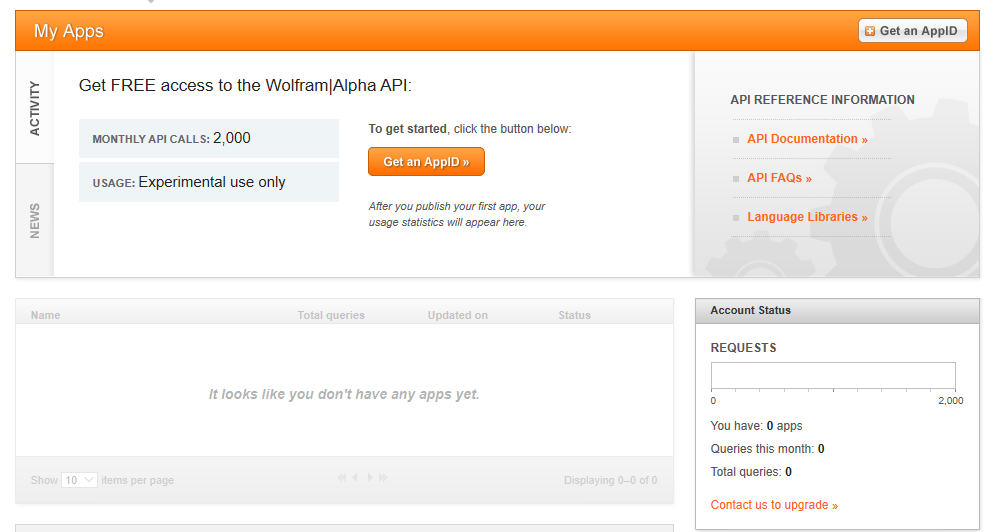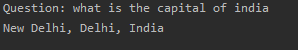Open In App
Related Articles
• Write an Interview Experience
• Python Projects – Beginner to Advanced

# Python | Create a simple assistant using Wolfram Alpha API.

The `Wolfram|Alpha Webservice API` provides a web-based API allowing the computational and presentation capabilities of Wolfram|Alpha to be integrated into web, mobile, desktop, and enterprise applications. `Wolfram Alpha` is an API which can compute expert-level answers using Wolfram’s algorithms, knowledgebase and AI technology. It is made possible by the Wolfram Language. This article tells how to create a simple assistant application in Python which can answer simple questions like the ones listed below.

```Input : What is the capital of India?
Output : New Delhi

Input : What is sin(30)?
Output : 0.5
```

Prerequisite: Basic understanding of python syntax and functions.

Getting API Id

1. Create a account at Wolfram alpha. The account can be created at the official website.3. Now you will see the homepage of the website. Head to the section in the top right corner where you see your email. In the drop down menu, select the My Apps (API) option.4. Click the Get an AppID button to get the id.5. In the next dialog box, give the app a suitable name and description.
6. Note down the APPID that appears in the next dialog box. This app id will be specific to the application.

Implementation

Make sure that `wolframalpha` python package is installed beforehand. It can be done by running the following command in the terminal or cmd –

```pip install wolframalpha
```

Below is the implementation

 `# Python program to ``# demonstrate creation of an``# assistant using wolf ram API`` ` `import` `wolframalpha`` ` `# Taking input from user``question ``=` `input``(``'Question: '``)`` ` `# App id obtained by the above steps``app_id ``=` `‘Your app_id’`` ` `# Instance of wolf ram alpha ``# client class``client ``=` `wolframalpha.Client(app_id)`` ` `# Stores the response from ``# wolf ram alpha``res ``=` `client.query(question)`` ` `# Includes only text from the response``answer ``=` `next``(res.results).text`` ` `print``(answer)`

Output: